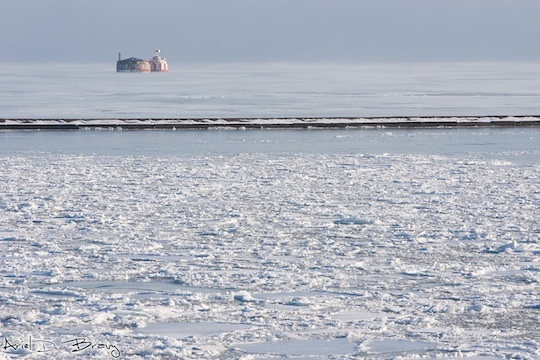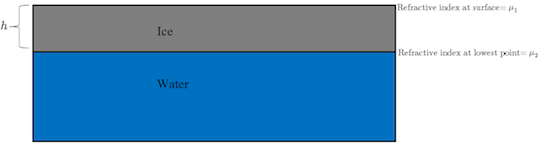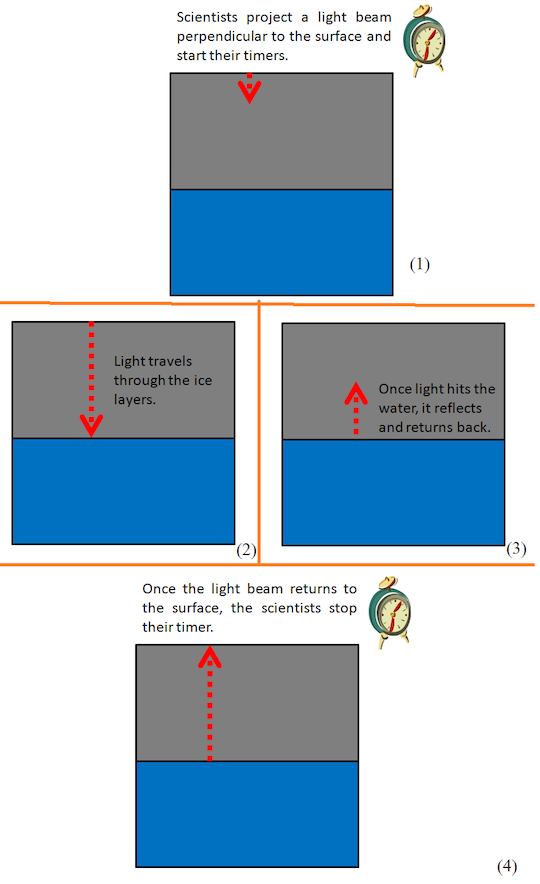# The Frozen LakeA group of scientists is given the job to determine the thickness of ice in a frozen lake. Ice being a good insulator of heat, one can find liquid water below a certain depth from the surface. However, the scientists don't have a meter-tape that is long enough to reach the water level, neither can they use a submarine to pierce through the ice. All they are given is a laser-pointer and a highly accurate timer.

Let's assume the ice in the lake is a transparent material, whose refractive index varies as a linear function of the depth. It is known that the refractive index at the surface is $\mu_1= 1.5$, and that at the lowest ice layer is $\mu_2= 3$.The scientists project a laser beam perpendicular to the surface and start their timer. The beam travels through the ice, hits the water, and reflects back. The scientists turn their timer off as soon as they see the laser beam return. It turns out that the time recorded on the timer is $T= 5 \times 10^{-6} \text{ seconds}$. What is the thickness $h$ of the ice in meters, rounded to the nearest integer?Details and assumptions

• Note that this experiment cannot be done in real life, as ice is an opaque material and water isn't a good reflector of light.
• The speed of light in vaccum is $c= 299, \ 792, \ 458 \text{ m/s}$.
• If $h$ in meters is of the form $k+0.5$ for some integer $k$, enter $k+1$ as your answer.
• The refractive index of ice varies as a linear function of depth simply means the refractive index at depth $d$ below the surface is given by $\mu_d= f(d)$, where $f$ is a linear polynomial.
• Reflex errors of the scientists are to be neglected.
×